How Cheenta works to ensure student success?
Explore the Back-Story

# PRMO 2016 Problem 2 | Number TheoryTry this beautiful interesting problem based on Number Theory from PRMO 2016 Problem 2.

## Number Theory Problem: PRMO 2016 Problem 2

The five digit number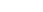is a perfect square. Find the value of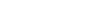.

### Key Concepts

Properties of Perfect Squares

Divisibility Rules of different numbers

Finding the square root of a number

## Suggested Book | Source | Answer

Challenge and Thrill of Pre College Mathematics

PRMO 2016

50

## Try with Hints

An odd perfect square is of the form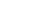Hence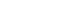Hence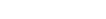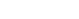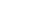Therefore the possible values of b are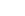So possible numbers are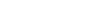Now check for the possible values of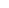and calculate the square root to check if it is a perfect square

The only valid solution is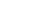Hence calculate the required expression.

## Subscribe to Cheenta at Youtube

Try this beautiful interesting problem based on Number Theory from PRMO 2016 Problem 2.

## Number Theory Problem: PRMO 2016 Problem 2

The five digit numberis a perfect square. Find the value of.

### Key Concepts

Properties of Perfect Squares

Divisibility Rules of different numbers

Finding the square root of a number

## Suggested Book | Source | Answer

Challenge and Thrill of Pre College Mathematics

PRMO 2016

50

## Try with Hints

An odd perfect square is of the formHenceHenceTherefore the possible values of b areSo possible numbers areNow check for the possible values ofand calculate the square root to check if it is a perfect square

The only valid solution isHence calculate the required expression.

## Subscribe to Cheenta at Youtube

This site uses Akismet to reduce spam. Learn how your comment data is processed.

### Knowledge Partner